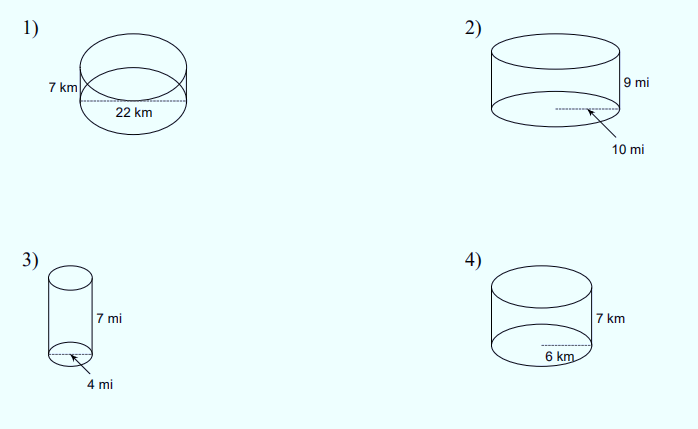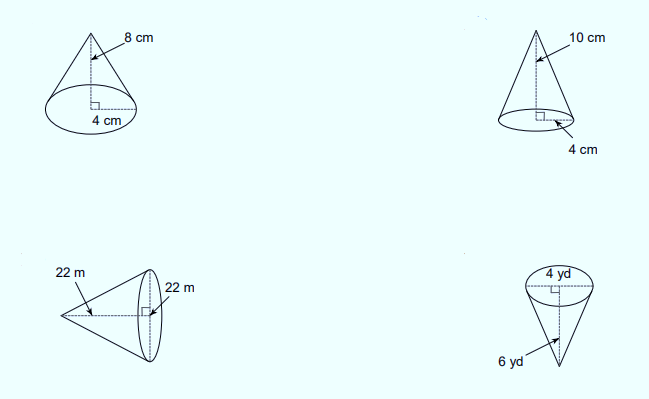# Volume of Cone and Cylinder Worksheet (with answer key + PDF)

The area or capacity of a cone is determined by its volume. A cone’s circular base tapers from a flat base to a point known as the apex or vertex in three dimensions. The density of a cylinder is determined by its volume, which represents how much material can be immersed in it or carried inside of it. The formula r2h, where r is the radius of the circular base and h is the height of the cylinder, determines the volume of a cylinder.

## What is the “Volume of Cone and Cylinder Worksheet”?

This worksheet will explore some of the Volumes of cylinders and cones.

## What is the volume of cylinders and cones?

A cylinder’s volume is determined by how many unit cubes (cubes of the same length) can fit inside of it. It is the area the cylinder takes up, just as any three-dimensional shape’s volume is the area it occupies. The area or volume that a cone takes up is referred to as its volume.

How will the “Volume of Cone and Cylinder Worksheet ” help you?

This worksheet will help the students to learn how to calculate the volume of a cylinder and cone and its formula using solved examples.

## Instructions on how to use the “Volume of Cone and Cylinder Worksheet.”

Study the concept and examples given and try to solve the given exercises below.

## Conclusion

The area contained by a 3D solid is its volume. Cubic units are used to measure volume. Formulas are written rules that relate quantities using mathematical symbols. You have already calculated the volumes of cubes and rectangular prisms using volume formulas. To calculate the volumes of cones, cylinders, and spheres, you can also use volume formulas.

If you have any inquiries or feedback, please let us know.

## Volume of Cone and Cylinder Worksheet (with answer key + PDF)

A cylinder is a solid shape with straight, parallel sides and a circular or oval base or cross-section. It has two circular bases joined by a curved surface and is a closed solid figure. A cylinder can be thought of as a limiting case of a prism.

The Volume of Cylinder Formula

V = A × h, where

• A = area of the base
• h = height

The volume of a Right Circular Cylinder

V = πr2h

Here,

• ‘r’ is the radius of the base (circle) of the cylinder
• ‘h’ is the height of the cylinder
• π is a constant whose value is either 22/7 (or) 3.142

The volume of an Oblique Cylinder

V = πr2h

• the volume (V) of an oblique cylinder whose base radius is ‘r’ and whose height is ‘h’.

The volume of an Elliptic Cylinder

V = πabh

Here,

• ‘a’ and ‘b’ are the radii of the base (ellipse) of the cylinder.
• ‘h’ is the height of the cylinder.
• π is a constant whose value is either 22/7 (or) 3.142.

Volume of a Right Circular Hollow Cylinder

V = π (R2 – r2) h

Here,

• ‘R’ is the base radius of the outside cylinder.
• ‘r’ is the base radius of the inside cylinder.
• ‘h’ is the height of the cylinder.
• π is a constant whose value is either 22/7 (or) 3.142.

Example of Volume Cylinder

A circle has an area of πr2. So, the formula for the volume of a cylinder is πr2h. Amari’s cake is a cylinder with a diameter of 44 cm and a height of 50 cm. What is the volume of the cake? The radius is half the diameter, so the radius is 22 cm.A cone is a strong, circular, three-dimensional shape. It has a surface that is curved. The perpendicular height is the distance from the base to the vertex. A cone can be categorized as either an oblique cone or a right circular cone. In contrast to an oblique cone, which has a vertex that is not vertically above the base center, the right circular cone has a vertex that is vertically above the center of the base. The volume of a cone is defined as the amount of space or capacity a cone occupies. The volume of the cone is measured in cubic units like cm3, m3, in3, etc.

The volume of Cone Formula

V = (1/3) πr2h

• If the radius of the base of the cone is “r” and the height of the cone is “h”

Volume of Cone with Height and Radius

V = (1/3) πr2h cubic units

Volume of Cone with Height and Diameter

V = (1/12) πd2h cubic units

The Volume of Cone with Slant Height

We know, h2 + r2 = L2
⇒ h = √ (L2 – r2)
where,

• h is the height of the cone,
• r is the radius of the base, and,
• L is the slant height of the cone

V = (1/3) πr2h = (1/3) πr2√ (L2 – r2).

Example of Volume Cone

The volume of space that a sphere can occupy is measured by its volume. If we take a circular disc, attach a string to its circumference, and rotate it along the string, we can draw a circle on a piece of paper. This gives us a sphere-like shape.

The volume of Cylinder = Volume of Cone + Volume of Sphere

⇒ Volume of Sphere = Volume of Cylinder – Volume of Cone
As we know, the volume of the cylinder = πr2h, and the volume of the cone = one-third of the volume of the cylinder = (1/3) πr2h
The volume of the Sphere = Volume of Cylinder – Volume of Cone

⇒ Volume of Sphere = πr2h – (1/3) πr2h = (2/3) πr2h
In this case, the height of cylinder = diameter of sphere = 2r
Hence, the volume of the sphere is (2/3) πr2h = (2/3) πr2(2r) = (4/3) πr3

## Worksheet

1. Find the volume of each figure.1.  For 5-8, find the volume of each cone.